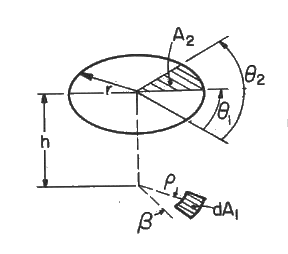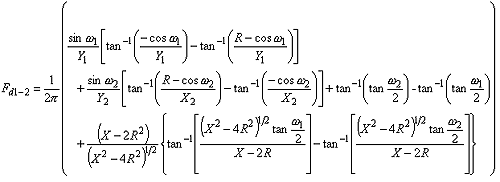>

# SECTION B Factors From Differential Elements to Finite Areas

## B-21: Plane element to sector of circular disk parallel to element.Definitions: H=h/r; R=r/r; Y=[H2+sin2ω]1/2; X=H2+R2+1; ω=cos-1[cos(θ-β)]

Governing equation:To download a copy of this equation in MS Word 97 Format, click HERE.